Next: Incoherent Pair Production Up: Physics and Numerical Methods Previous: Enhancement factor of the

## Coherent Pair Creation

When a high energy photon goes through a strong transverse field, it can create a real electron-positron pair. This process is known as `coherent pair creation' and is characterized by the parameter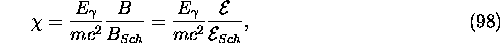where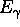is the energy of the initial photon. (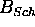and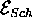are defined in eq.(84).) The probability of the process is exponentially small (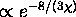) when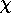is small.

Let us denote the energy and polarization vector of initial photon and final positron/ electron by,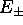(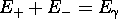),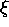, and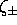. The transition rate is obtained by the following replacement in the formula (92):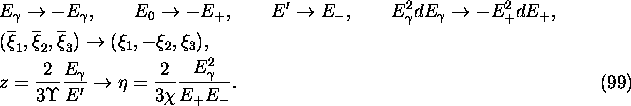Ignoring the terms related to the polarization correlation between the final electron and positron, we obtain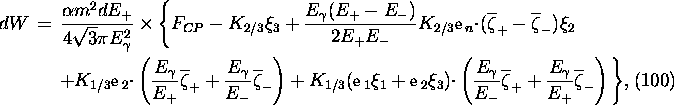where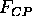is defined as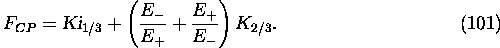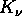is the modified Bessel function and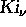is defined in eq.(87). Their arguments are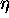defined in eq.(99). The transition rate summed over the final polarization is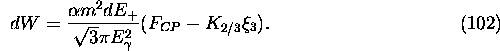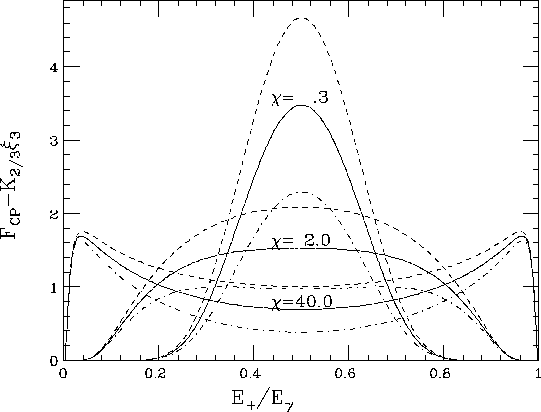Figure 9: Function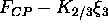for three values of. The solid, dotdash and dashed curves are for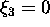, 1, -1, respectively. The curves forare normalized such that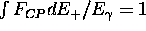, and those for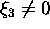are drawn with the same scale as the correspondingcurves.

The functionis plotted in Fig.9 as a function of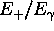.

1ex

The total rate for givenis approximately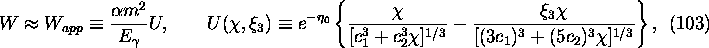where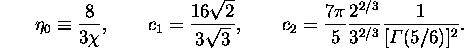In order to make the spectrum function flatter, CAIN introduces the variable y (-1<y<1) instead of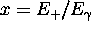(0<x<1):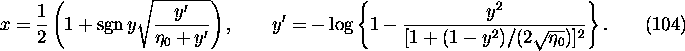The spectrum function with respect to y then becomes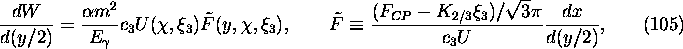where y/2 is used because the range of y is 2. The constant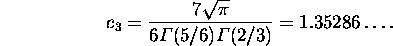is chosen so that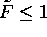for any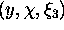.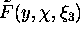is plotted in Fig.10.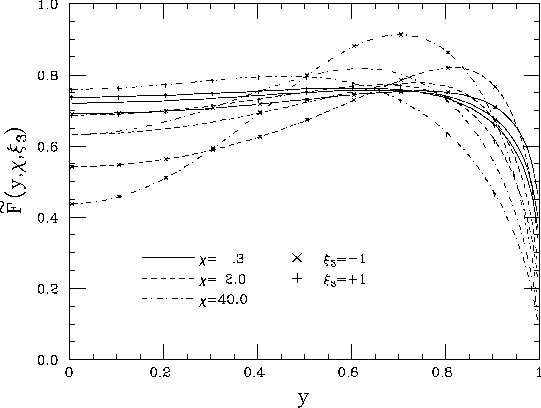Figure 10: Functionas a function of y for three values of=0.3, 2, 40 and for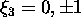. The parameteris indicated by the line mode and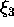is by crosses (no cross for).

Now, the event generation goes as

(1)
Computeand reject if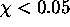(the rate is too small).
(2)
Generate a uniform random number 0<p<1 and compute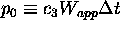.
(3)
Reject if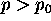.
(4)
Generate another uniform random numbers 0<q<1 and -1<y<1 and compute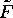. (Instead of q, one can also use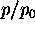.)
(5)
Reject if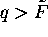.
(6)
Accept and compute x from eq.(104) and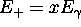,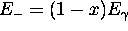.
(7)
Compute the final polarization of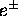from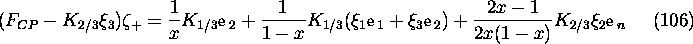The formula for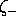is obtained by replacing x by 1-x.
(8)
In the case of rejection, the polarization of the photon should be changed according to eq.(12):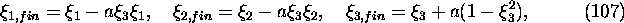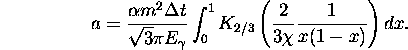The error of the formula (103) does not cause any inaccuracy of event generation (but causes inefficiency).Next: Incoherent Pair Production Up: Physics and Numerical Methods Previous: Enhancement factor of the

Toshiaki Tauchi
Thu Dec 3 17:27:26 JST 1998